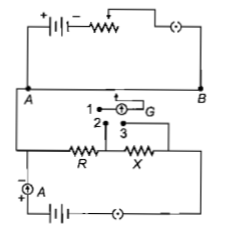A potentiometer circuit is set up as shown.The potential gradient across the potentiometer wire, is k volt/cm and the ammeter, present in the circuit, reads 1.0A when two way key is switched off.The balance points, when the key is switched off.The balance points, when the key between the terminals (i) 1 and 2 (ii) 1and 3, is plugged in, are found to be at lengths  respectively.The magnitudes, of the resistors R and X, in ohm, are then, equal, respectively, to(a)

(b)

(c)

(d)